# Implementing Apriori algorithm in Python

Prerequisites: Apriori Algorithm

Apriori Algorithm is a Machine Learning algorithm which is used to gain insight into the structured relationships between different items involved. The most prominent practical application of the algorithm is to recommend products based on the products already present in the user’s cart. Walmart especially has made great use of the algorithm in suggesting products to it’s users.

Dataset : Groceries data
Implementation of algorithm in Python:
Step 1: Importing the required libraries

 `import` `numpy as np ` `import` `pandas as pd ` `from` `mlxtend.frequent_patterns ``import` `apriori, association_rules `

 `# Changing the working location to the location of the file ` `cd C:\Users\Dev\Desktop\Kaggle\Apriori Algorithm ` ` `  `# Loading the Data ` `data ``=` `pd.read_excel(``'Online_Retail.xlsx'``) ` `data.head() `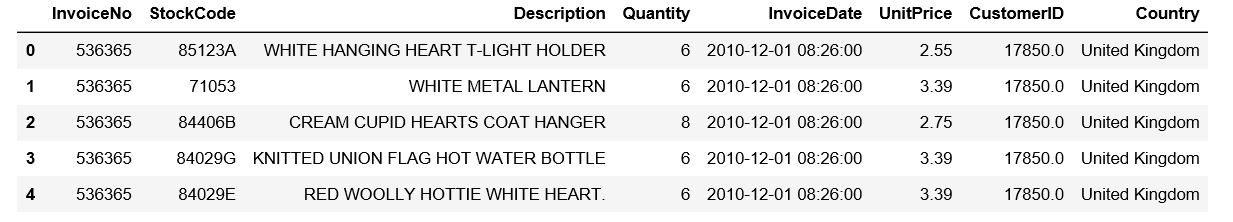`# Exploring the columns of the data ` `data.columns `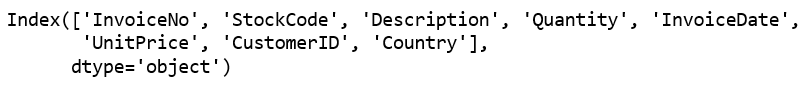`# Exploring the different regions of transactions ` `data.Country.unique() `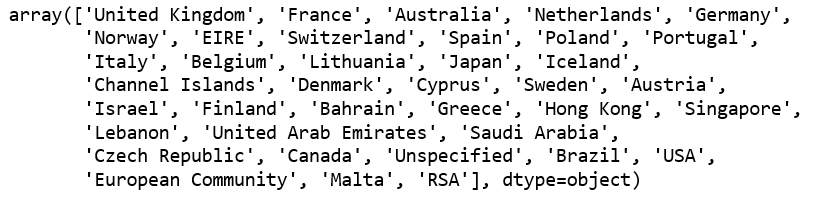Step 3: Cleaning the Data

 `# Stripping extra spaces in the description ` `data[``'Description'``] ``=` `data[``'Description'``].``str``.strip() ` ` `  `# Dropping the rows without any invoice number ` `data.dropna(axis ``=` `0``, subset ``=``[``'InvoiceNo'``], inplace ``=` `True``) ` `data[``'InvoiceNo'``] ``=` `data[``'InvoiceNo'``].astype(``'str'``) ` ` `  `# Dropping all transactions which were done on credit ` `data ``=` `data[~data[``'InvoiceNo'``].``str``.contains(``'C'``)] `

Step 4: Splitting the data according to the region of transaction

 `# Transactions done in France ` `basket_France ``=` `(data[data[``'Country'``] ``=``=``"France"``] ` `          ``.groupby([``'InvoiceNo'``, ``'Description'``])[``'Quantity'``] ` `          ``.``sum``().unstack().reset_index().fillna(``0``) ` `          ``.set_index(``'InvoiceNo'``)) ` ` `  `# Transactions done in the United Kingdom ` `basket_UK ``=` `(data[data[``'Country'``] ``=``=``"United Kingdom"``] ` `          ``.groupby([``'InvoiceNo'``, ``'Description'``])[``'Quantity'``] ` `          ``.``sum``().unstack().reset_index().fillna(``0``) ` `          ``.set_index(``'InvoiceNo'``)) ` ` `  `# Transactions done in Portugal ` `basket_Por ``=` `(data[data[``'Country'``] ``=``=``"Portugal"``] ` `          ``.groupby([``'InvoiceNo'``, ``'Description'``])[``'Quantity'``] ` `          ``.``sum``().unstack().reset_index().fillna(``0``) ` `          ``.set_index(``'InvoiceNo'``)) ` ` `  `basket_Sweden ``=` `(data[data[``'Country'``] ``=``=``"Sweden"``] ` `          ``.groupby([``'InvoiceNo'``, ``'Description'``])[``'Quantity'``] ` `          ``.``sum``().unstack().reset_index().fillna(``0``) ` `          ``.set_index(``'InvoiceNo'``)) `

Step 5: Hot encoding the Data

 `# Defining the hot encoding function to make the data suitable  ` `# for the concerned libraries ` `def` `hot_encode(x): ` `    ``if``(x<``=` `0``): ` `        ``return` `0` `    ``if``(x>``=` `1``): ` `        ``return` `1` ` `  `# Encoding the datasets ` `basket_encoded ``=` `basket_France.applymap(hot_encode) ` `basket_France ``=` `basket_encoded ` ` `  `basket_encoded ``=` `basket_UK.applymap(hot_encode) ` `basket_UK ``=` `basket_encoded ` ` `  `basket_encoded ``=` `basket_Por.applymap(hot_encode) ` `basket_Por ``=` `basket_encoded ` ` `  `basket_encoded ``=` `basket_Sweden.applymap(hot_encode) ` `basket_Sweden ``=` `basket_encoded `

Step 6: Buliding the models and analyzing the results

a) France:

 `# Building the model ` `frq_items ``=` `apriori(basket_France, min_support ``=` `0.05``, use_colnames ``=` `True``) ` ` `  `# Collecting the inferred rules in a dataframe ` `rules ``=` `association_rules(frq_items, metric ``=``"lift"``, min_threshold ``=` `1``) ` `rules ``=` `rules.sort_values([``'confidence'``, ``'lift'``], ascending ``=``[``False``, ``False``]) ` `print``(rules.head()) `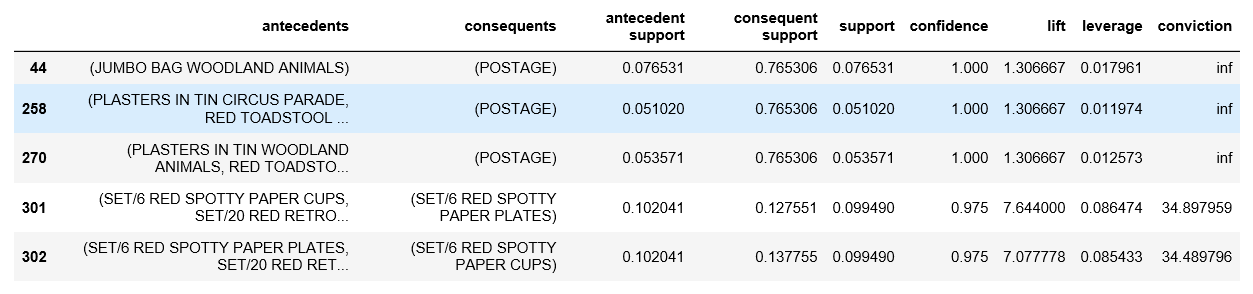From the above output, it can be seen that paper cups and paper and plates are bought together in France. This is because the French have a culture of having a get-together with their friends and family atleast once a week. Also, since the French government has banned the use of plastic in the country, the people have to purchase the paper -based alternatives.

b) United Kingdom:

 `frq_items ``=` `apriori(basket_UK, min_support ``=` `0.01``, use_colnames ``=` `True``) ` `rules ``=` `association_rules(frq_items, metric ``=``"lift"``, min_threshold ``=` `1``) ` `rules ``=` `rules.sort_values([``'confidence'``, ``'lift'``], ascending ``=``[``False``, ``False``]) ` `print``(rules.head()) `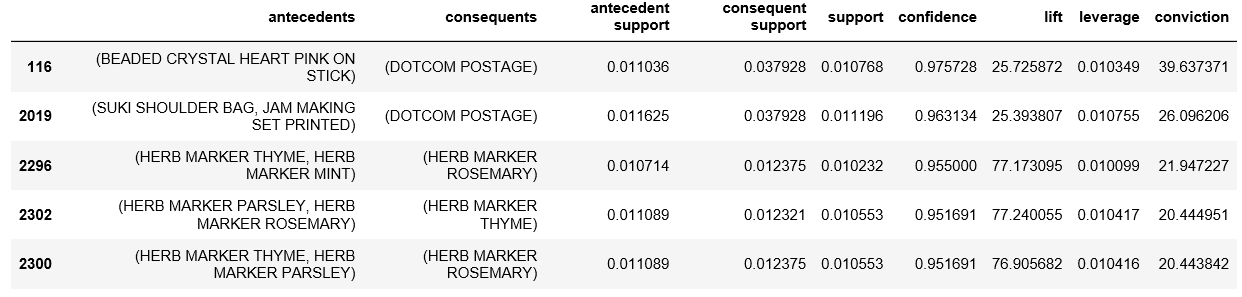If the rules for British transactions are analyzed a little deeper, it is seen that the British people buy different coloured tea-plates together. A reason behind this may be because typically the British enjoy tea very much and often collect different coloured tea-plates for different ocassions.

c) Portugal:

 `frq_items ``=` `apriori(basket_Por, min_support ``=` `0.05``, use_colnames ``=` `True``) ` `rules ``=` `association_rules(frq_items, metric ``=``"lift"``, min_threshold ``=` `1``) ` `rules ``=` `rules.sort_values([``'confidence'``, ``'lift'``], ascending ``=``[``False``, ``False``]) ` `print``(rules.head()) `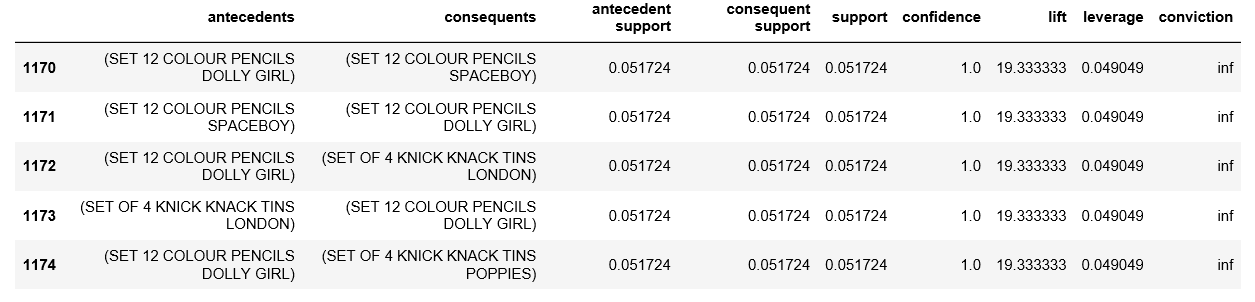On analyzing the association rules for Portuguese transactions, it is observed that Tiffin sets (Knick Knack Tins) and colour pencils. These two products typically belong to a primary school going kid. These two products are required by children in school to carry their lunch and for creative work respectively and hence are logically make sense to be paired together.

d) Sweden:

 `frq_items ``=` `apriori(basket_Sweden, min_support ``=` `0.05``, use_colnames ``=` `True``) ` `rules ``=` `association_rules(frq_items, metric ``=``"lift"``, min_threshold ``=` `1``) ` `rules ``=` `rules.sort_values([``'confidence'``, ``'lift'``], ascending ``=``[``False``, ``False``]) ` `print``(rules.head()) `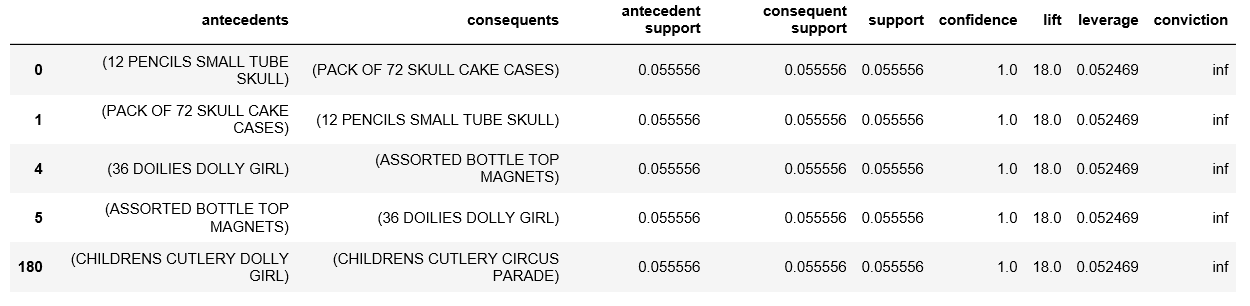On analyzing the above rules, it is found that boys’ and girls’ cutlery are paired together. This makes practical sense because when a parent goes shopping for cutlery for his/her children, he/she would want the product to be a little customized according to the kid’s wishes.

My Personal Notes arrow_drop_upCheck out this Author's contributed articles.

If you like GeeksforGeeks and would like to contribute, you can also write an article using contribute.geeksforgeeks.org or mail your article to contribute@geeksforgeeks.org. See your article appearing on the GeeksforGeeks main page and help other Geeks.

Please Improve this article if you find anything incorrect by clicking on the "Improve Article" button below.

Article Tags :
Practice Tags :

4

Please write to us at contribute@geeksforgeeks.org to report any issue with the above content.